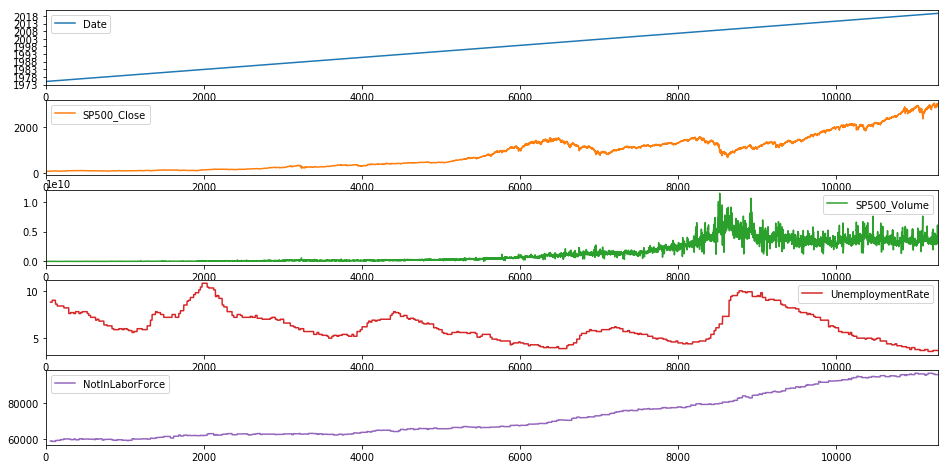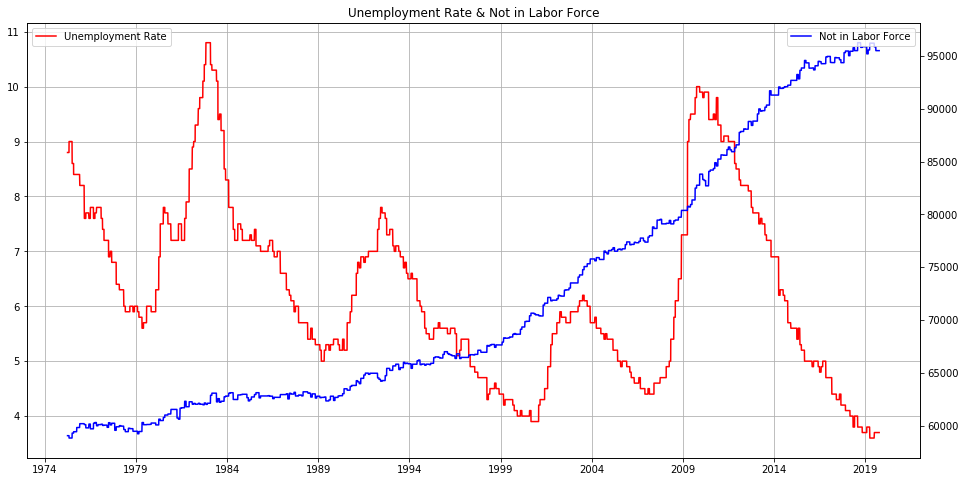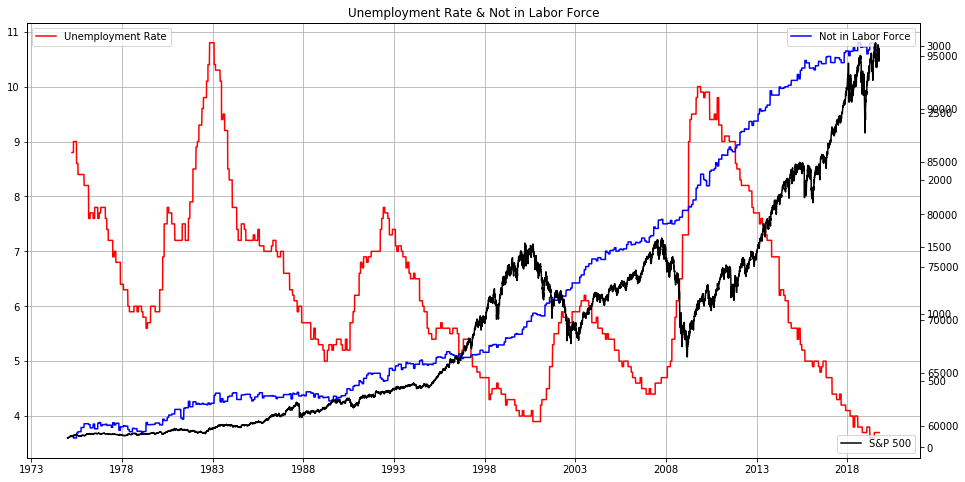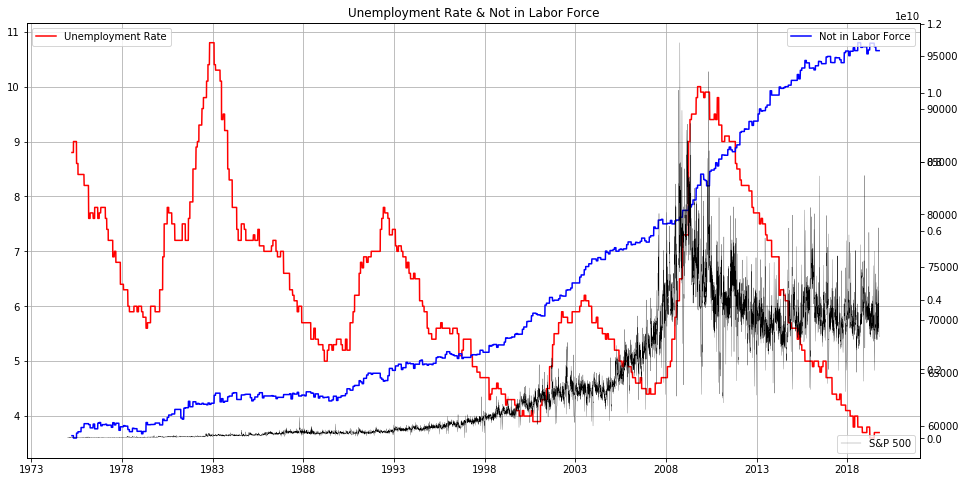### Get the "Applied Data Science Edge"!Fundamental Market Analysis with Python - Find Your Own Answers On What Is Going on in the Financial Markets

### Hot off the Press!My New Book: "The Little Book of Fundamental Analysis: Hands-On Market Analysis with Python" is Out!

### Grow Your Web Brand, Visibility & Traffic Organically5 Years of amunategui.github.Io and the Lessons I Learned from Growing My Online Community from the Ground Up### Introduction

We are seeing some of the lowest unemployment in 50 years yet the highest drop in the labor force. Let's investigate this ourselves by finding the correct data sets and survey results to shed light on this weird phenomenon.

If you liked it, please share it:

### Code

ViralML-Unemployment-Rate-and-Not-Labor-Force-Hands-On-with-Python
In :
from IPython.display import Image
Image(filename='viralml-book.png')

Out:# "50 year low in unemployment rate with nearly 100 million not in the labor force🤔" -- @NorthmanTrader¶

Good read - Persons not in the labor force by desire and availability for work, age, and sex:

https://www.bls.gov/web/empsit/cpseea38.htm

## Data Needed to Follow Along¶

### From the Federal Reserve Bank of St. Louis¶

#### Not in Labor Force (LNS15000000)¶

https://fred.stlouisfed.org/series/LNS15000000

The series comes from the 'Current Population Survey (Household Survey)'

#### Unemployment Rate (UNRATE)¶

https://fred.stlouisfed.org/series/UNRATE

The unemployment rate represents the number of unemployed as a percentage of the labor force. Labor force data are restricted to people 16 years of age and older, who currently reside in 1 of the 50 states or the District of Columbia, who do not reside in institutions (e.g., penal and mental facilities, homes for the aged), and who are not on active duty in the Armed Forces.

### From the Yahoo Finance¶

#### S&P 500 (^GSPC)¶

https://finance.yahoo.com/quote/%5EGSPC

In :
%matplotlib inline
import matplotlib
import matplotlib.pyplot as plt
import io, base64, os, json, re
import pandas as pd
import numpy as np
import datetime
import warnings
warnings.filterwarnings('ignore')

path_to_market_data = '/Users/manuel/Documents/financial-research/market-data/2019-10-07/'


In :
# Load Not in Labor Force data
not_labor_force_df.columns = ['Date', 'NotInLaborForce']
not_labor_force_df['Date'] = pd.to_datetime(not_labor_force_df['Date'])
print(np.min(not_labor_force_df['Date'] ),np.max(not_labor_force_df['Date'] ))
not_labor_force_df = not_labor_force_df.sort_values('Date', ascending=True) # sort in ascending date order
nil()
ot_labor_force_df.ta

1975-01-01 00:00:00 2019-09-01 00:00:00

Out:
Date NotInLaborForce
532 2019-05-01 96215
533 2019-06-01 96057
534 2019-07-01 95874
535 2019-08-01 95510
536 2019-09-01 95599
In :
# Load Unemployment Rate data
unemployment_df.columns = ['Date', 'UnemploymentRate']
unemployment_df['Date'] = pd.to_datetime(unemployment_df['Date'])
print(np.min(unemployment_df['Date'] ),np.max(unemployment_df['Date'] ))
unemployment_df = unemployment_df.sort_values('Date', ascending=True) # sort in ascending date order
unemployment_df.tail()

1948-01-01 00:00:00 2019-09-01 00:00:00

Out:
Date UnemploymentRate
856 2019-05-01 3.6
857 2019-06-01 3.7
858 2019-07-01 3.7
859 2019-08-01 3.7
860 2019-09-01 3.5
In :
# Load S&P 500 Index data
gspc_df['Date'] = pd.to_datetime(gspc_df['Date'])
gspc_df = gspc_df[['Date', 'Adj Close',  'Volume']]
gspc_df.columns = ['Date', 'SP500_Close', 'SP500_Volume']
gspc_df = gspc_df.sort_values('Date', ascending=True)
print(min(gspc_df['Date']), max(gspc_df['Date']))
print(gspc_df.shape)
gspc_df.tail()

1927-12-30 00:00:00 2019-10-07 00:00:00
(23050, 3)

Out:
Date SP500_Close SP500_Volume
23045 2019-10-01 2940.250000 3558040000
23046 2019-10-02 2887.610107 3912520000
23047 2019-10-03 2910.629883 3503640000
23048 2019-10-04 2952.010010 2990830000
23049 2019-10-07 2938.790039 2940140000

## Join all data together¶

In :
cut_off_date = '1975-01-01'
tmp_not_labor_force_df = not_labor_force_df.copy()
tmp_not_labor_force_df = tmp_not_labor_force_df[tmp_not_labor_force_df['Date'] >= cut_off_date]

tmp_unemployment_df = unemployment_df.copy()
tmp_unemployment_df = tmp_unemployment_df[tmp_unemployment_df['Date'] >= cut_off_date]

tmp_gspc_df = gspc_df.copy()
tmp_gspc_df = tmp_gspc_df[tmp_gspc_df['Date'] >= cut_off_date]

# join into single data frame
together_df = pd.merge(tmp_gspc_df,
tmp_unemployment_df, on= ['Date'], how='left')
together_df = pd.merge(together_df,
tmp_not_labor_force_df, on= ['Date'], how='left')

# last valid observation forward
together_df = together_df.fillna(method='ffill')

together_df.tail()

Out:
Date SP500_Close SP500_Volume UnemploymentRate NotInLaborForce
11286 2019-10-01 2940.250000 3558040000 3.7 95510.0
11287 2019-10-02 2887.610107 3912520000 3.7 95510.0
11288 2019-10-03 2910.629883 3503640000 3.7 95510.0
11289 2019-10-04 2952.010010 2990830000 3.7 95510.0
11290 2019-10-07 2938.790039 2940140000 3.7 95510.0

## Plot the results¶

In :
fig, ax = plt.subplots(figsize=(16, 8))
together_df.plot(subplots=True, ax=ax)

Out:
array([<matplotlib.axes._subplots.AxesSubplot object at 0x11f639e80>,
<matplotlib.axes._subplots.AxesSubplot object at 0x11f900d30>,
<matplotlib.axes._subplots.AxesSubplot object at 0x11f935208>,
<matplotlib.axes._subplots.AxesSubplot object at 0x11f95c898>,
<matplotlib.axes._subplots.AxesSubplot object at 0x11f9cb358>],
dtype=object)In :
fig, ax = plt.subplots(figsize=(16, 8))
plt.plot(together_df['Date'],
together_df['UnemploymentRate'], color='red', label='Unemployment Rate')
plt.grid()
plt.title("Unemployment Rate & Not in Labor Force")
plt.legend(loc='upper left')

ax.twinx()
plt.plot(together_df['Date'],
together_df['NotInLaborForce'] , color='blue', label='Not in Labor Force')

plt.legend(loc='upper right')

plt.show()In :
fig, ax = plt.subplots(figsize=(16, 8))
plt.plot(together_df['Date'],
together_df['UnemploymentRate'], color='red', label='Unemployment Rate')
plt.grid()
plt.title("Unemployment Rate & Not in Labor Force")
plt.legend(loc='upper left')

ax.twinx()
plt.plot(together_df['Date'],
together_df['NotInLaborForce'] , color='blue', label='Not in Labor Force')

plt.legend(loc='upper right')

ax.twinx()
plt.plot(together_df['Date'],
together_df['SP500_Close'] , color='black', label="S&P 500")

plt.legend(loc='lower right')

plt.show()In :
fig, ax = plt.subplots(figsize=(16, 8))
plt.plot(together_df['Date'],
together_df['UnemploymentRate'], color='red', label='Unemployment Rate')
plt.grid()
plt.title("Unemployment Rate & Not in Labor Force")
plt.legend(loc='upper left')

ax.twinx()
plt.plot(together_df['Date'],
together_df['NotInLaborForce'] , color='blue', label='Not in Labor Force')

plt.legend(loc='upper right')

ax.twinx()
plt.plot(together_df['Date'],
together_df['SP500_Volume'] , color='black', linewidth=0.2, label="S&P 500")

plt.legend(loc='lower right')

plt.show()### Show Notes

(pardon typos and formatting -
these are the notes I use to make the videos)

We are seeing some of the lowest unemployment in 50 years yet the highest drop in the labor force. Let's investigate this ourselves by finding the correct data sets and survey results to shed light on this weird phenomenon.